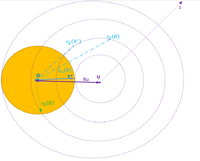# INTEGRATION LIMITS OF A CIRCLE WITH A CIRCULAR VACANCY

#### Maurilio

##### New member
Hi everyone!
I am trying to express the integration limits of the generic region defined by the dotted purple circumferences (of radius Ru-R*<z<Ru+R*), excluding the yellow circle, with respect to the system of polar coordinates (θ, r) centered in O.
Each circumference is identified by the values of r_m(θ) and r_X(θ), where of course -θ*(z)<θ(z)<θ*(z), r_m(θ)=0 if z>=Ru, θ*(Ru)=pi/2, and θ*(z)=pi if z>Ru. The mathematical expressions of the functions θ*(z), r_m(θ), and r_X(θ) are known.

#### Attachments

• 62.1 KB Views: 1

#### Subhotosh Khan

##### Super Moderator
Staff member
Hi everyone!
I am trying to express the integration limits of the generic region defined by the dotted purple circumferences (of radius Ru-R*<z<Ru+R*), excluding the yellow circle, with respect to the system of polar coordinates (θ, r) centered in O.
Each circumference is identified by the values of r_m(θ) and r_X(θ), where of course -θ*(z)<θ(z)<θ*(z), r_m(θ)=0 if z>=Ru, θ*(Ru)=pi/2, and θ*(z)=pi if z>Ru. The mathematical expressions of the functions θ*(z), r_m(θ), and r_X(θ) are known.